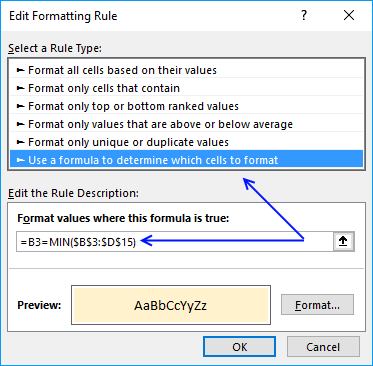Author: Oscar Cronquist Article last updated on January 09, 2019

The image above demonstrates a conditional formatting formula that highlights records that only exist in one table. There are two tables in this example: List 1 and List 2.

If you are looking for a conditional formatting formula that highlights records shared by both tables: Compare tables: Highlight common records. There is also an article that demonstrates how to filter records not in both tables.

The conditional formatting formula does not work with cell references outside the current sheet so we need to rely on named ranges for this to work.

### Create named ranges

1. Select A2:A13 on sheet "List 2"
2. Type Year in Name Box
3. Press Enter

Repeat with remaining ranges:
Sheet: List 2 , Range:B2:B13, Name: Asset
Sheet: List 2 , Range:C2:C13, Name: Cost
Sheet: List 1 , Range:A2:A11, Name: Year1
Sheet: List 1 , Range:B2:B11, Name: Asset1
Sheet: List 1 , Range:C2:C11, Name: Cost1

### Highlight records existing in only one list

How to apply conditional formatting formula:

Sheet: List 1

1. Select cells A2:C11 (Sheet: List 1)
2. Click "Home" tab
3. Click "Conditional Formatting" button
4. Click "New Rule.."
5. Click "Use a formula to determine which cells to format"
6. Type =COUNTIFS(Year, \$A2, Asset, \$B2, Cost, \$C2)=0 in "Format values where this formula is TRUE" window.(The formula displayed in the image above is not used in this article)
7. Click "Format.." button
8. Click "Fill" tab
9. Select a color for highlighting cells.
10. Click "Ok"
11. Click "Ok"
12. Click "Ok"

Sheet: List 2

1. Select cells A2:C13 (Sheet: List 2)
2. Click "Home" tab
3. Click "Conditional Formatting" button
4. Click "New Rule.."
5. Click "Use a formula to determine which cells to format"
6. Type =COUNTIFS(Year1, \$A2, Asset1, \$B2, Cost1, \$C2)=0 in "Format values where this formula is TRUE" window.(The formula displayed in the image above is not used in this article)
7. Click "Format.." button
8. Click "Fill" tab
9. Select a color for highlighting cells.
10. Click "Ok"
11. Click "Ok"
12. Click "Ok"

### Highlight records existing in only one list, excel 2003

The COUNTIFS function was introduced in Excel 2007 and if you have an earlier version you need another formula shown below. The COUNTIF functions returns arrays that are multiplied, the SUMPRODUCT function then adds the numbers and returns a total.

Sheet: List 1

Conditional formatting formula:

=SUMPRODUCT(COUNTIF(\$A2, Year)*COUNTIF(\$B2, Asset)*COUNTIF(\$C2, Cost))=0

Sheet: List 2

Conditional formatting formula:

=SUMPRODUCT(COUNTIF(\$A2, Year1)*COUNTIF(\$B2, Asset1)*COUNTIF(\$C2, Cost1))=0

Sheet: List 1

Sheet: List 2